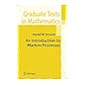Normal view

# An Introduction to Markov Processes

Material type:Computer fileLanguage: English Series: Graduate Texts in Mathematics ; 230Publication details: 2014 Edition: 2nd ed. 2014Description: Online-Ressource (XVII, 203 p) online resourceISBN: 978-3540234517; 9783642405228DDC classification: 519.2 LOC classification: QA273.A1-274.9QA274-274.9Other classification: SK 820 | mat Online resources: Volltext | Zentralblatt MATH Inhaltstext
Contents:
PrefaceRandom Walks, a Good Place to Begin -- Doeblin's Theory for Markov Chains -- Stationary Probabilities -- More about the Ergodic Theory of Markov Chains -- Markov Processes in Continuous Time -- Reversible Markov Processes -- A minimal Introduction to Measure Theory -- Notation -- References -- Index..
Summary: This book provides a rigorous but elementary introduction to the theory of Markov Processes on a countable state space. It should be accessible to students with a solid undergraduate background in mathematics, including students from engineering, economics, physics, and biology. Topics covered are: Doeblin's theory, general ergodic properties, and continuous time processes. Applications are dispersed throughout the book. In addition, a whole chapter is devoted to reversible processes and the use of their associated Dirichlet forms to estimate the rate of convergence to equilibrium. These results are then applied to the analysis of the Metropolis (a.k.a simulated annealing) algorithm. The corrected and enlarged 2nd edition contains a new chapter in which the author develops computational methods for Markov chains on a finite state space. Most intriguing is the section with a new technique for computing stationary measures, which is applied to derivations of Wilson's algorithm and Kirchoff's formula for spanning trees in a connected graph
Tags from this library: No tags from this library for this title.
Star ratingsAverage rating: 0.0 (0 votes)
Holdings
Item type Current library Collection Call number Status Date due Barcode Item holdsBook Books at groups
Erdős Group Not for loan
Total holds: 0

PrefaceRandom Walks, a Good Place to Begin -- Doeblin's Theory for Markov Chains -- Stationary Probabilities -- More about the Ergodic Theory of Markov Chains -- Markov Processes in Continuous Time -- Reversible Markov Processes -- A minimal Introduction to Measure Theory -- Notation -- References -- Index..

This book provides a rigorous but elementary introduction to the theory of Markov Processes on a countable state space. It should be accessible to students with a solid undergraduate background in mathematics, including students from engineering, economics, physics, and biology. Topics covered are: Doeblin's theory, general ergodic properties, and continuous time processes. Applications are dispersed throughout the book. In addition, a whole chapter is devoted to reversible processes and the use of their associated Dirichlet forms to estimate the rate of convergence to equilibrium. These results are then applied to the analysis of the Metropolis (a.k.a simulated annealing) algorithm. The corrected and enlarged 2nd edition contains a new chapter in which the author develops computational methods for Markov chains on a finite state space. Most intriguing is the section with a new technique for computing stationary measures, which is applied to derivations of Wilson's algorithm and Kirchoff's formula for spanning trees in a connected graph

Online edition Springer eBook Collection. Mathematics and Statistics

## Other editions of this workAn Introduction to Markov Processes (Graduate Texts in Mathematics). by Stroock, Daniel W. ©2005
Share